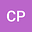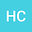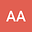BayClump: Bayesian Calibration and Temperature Reconstructions for Clumped Isotope Thermometry
•••• +5
Cristian Román PalaciosUniversity of California -Los Angeles, University of California -Los Angeles

Corresponding Author:cromanpa94@email.arizona.edu

Author ProfileHannah Carroll
University of California -Los Angeles, University of California -Los Angeles
Author ProfileRandy Flores
University of California -Los Angeles, University of California -Los Angeles
Author ProfileQuan Gan
University of Arizona
Author Profile## Abstract

Carbonate clumped isotope thermometry (Δ_47) is a temperature proxy that is becoming more widely used in the geosciences. Most calibration studies have used ordinary least squares linear regressions or York models to describe the relationship between Δ_47 and temperature. However, Bayesian models have not yet been explored for clumped isotopes. There also has not yet been a comprehensive study assessing the performance of commonly used regression models in the field. Here, we use simulated datasets to compare the performance of seven regression models, three of which are new and fit using a Bayesian framework. While Bayesian and non-Bayesian ordinary least squares linear regression models show the best overall accuracy for calibrations, Bayesian models outperform other models in terms of precision, especially if datasets are sufficiently large (>50 data points). For temperature reconstructions where a given regression model is applied to predict temperature from Δ_47), Bayesian and non-Bayesian models show variable performance advantages depending on the the structure of errors in the calibration dataset. Overall, our analyses suggest that the advantages of using Bayesian models for calibrating and reconstructing temperatures using clumped isotope paleothermometry are realized through the use of large calibration datasets (>50 data points). When used with large datasets, Bayesian regressions are expected to substantially improve the accuracy and precision of (i) calibration parameter estimates and (ii) temperature reconstructions (e.g., typically improving precision by at least a factor of two). We implement our comparative framework into a new web-based interface, BayClump. This data tool should increase reproducibility by enabling access to the different Bayesian and non-Bayesian regression models. Finally, we utilize BayClump with three published datasets to examine precision and accuracy in regression parameters and reconstructed temperatures. We show that BayClump yields similarly accurate results to published studies. However, the use of BayClump generally produces temperature reconstructions with meaningful reductions in temperature uncertainty, as demonstrated through reanalysis of data from a Late Miocene hominoids site in Yunnan, China.